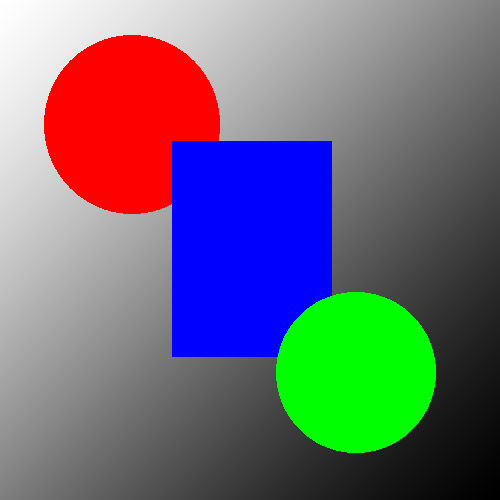# Adjust the Contrast of an Image in C#Adjusting the contrast of an image in C# is an easy operation because it can be done on a single pixel at a time. We only need to know 2 formulas to calculate a new pixel color based on an old pixel color, and they’re very easy to implement.

• contrast = ((100.0 + T) / 100.0)2
• newRed = ( ( ( (oldRed / 255.0) – 0.5) * contrast) + 0.5) * 255.0

Here’s a simple function that implements this formula for each pixel in a Bitmap object.

``````// this code relies on the LockedBitmap class
// threshold should be a value between -100 and 100
private static void SetContrast(Bitmap bmp, int threshold)
{
var lockedBitmap = new LockBitmap(bmp);
lockedBitmap.LockBits();

var contrast = Math.Pow((100.0 + threshold) / 100.0, 2);

for (int y = 0; y < lockedBitmap.Height; y++)
{
for (int x = 0; x < lockedBitmap.Width; x++)
{
var oldColor = lockedBitmap.GetPixel(x, y);
var red = ((((oldColor.R / 255.0) - 0.5) * contrast) + 0.5) * 255.0;
var green = ((((oldColor.G / 255.0) - 0.5) * contrast) + 0.5) * 255.0;
var blue = ((((oldColor.B / 255.0) - 0.5) * contrast) + 0.5) * 255.0;
if (red > 255) red = 255;
if (red < 0) red = 0;
if (green > 255) green = 255;
if (green < 0) green = 0;
if (blue > 255) blue = 255;
if (blue < 0) blue = 0;

var newColor = Color.FromArgb(oldColor.A, (int)red, (int)green, (int)blue);
lockedBitmap.SetPixel(x, y, newColor);
}
}
lockedBitmap.UnlockBits();
}``````

Here is a sample image.And here is that same image with the contrast adjusted to -50.And here is that same image with the contrast adjusted up to 50.#### How it Works

This block of code iterates over the width and height of a locked Bitmap object looking at each pixel one at a time. For each pixel it calculates a new Color object based on the formula provided above. You call this function with a threshold between -100 and 100.

#### The LockedBitmap Class

You need to have the LockedBitmap class to make this example work. You can read about it on the How to Open an Image in C# guide.

### Other C# Image Processing Guides

Be sure to check out some of our other C# Image Processing Guides for related guides.

This site uses Akismet to reduce spam. Learn how your comment data is processed.